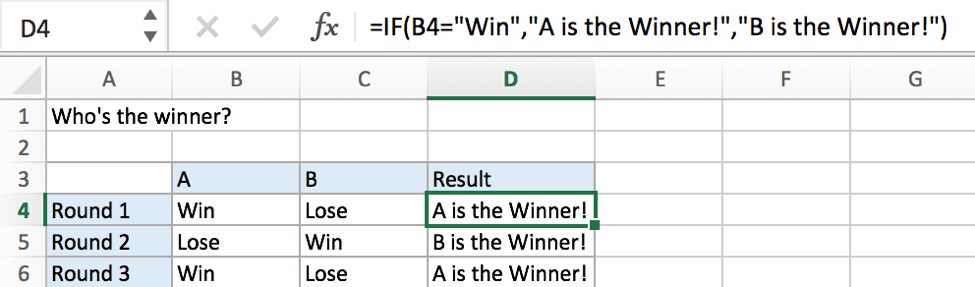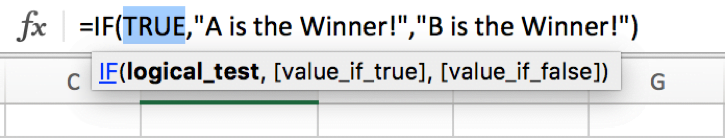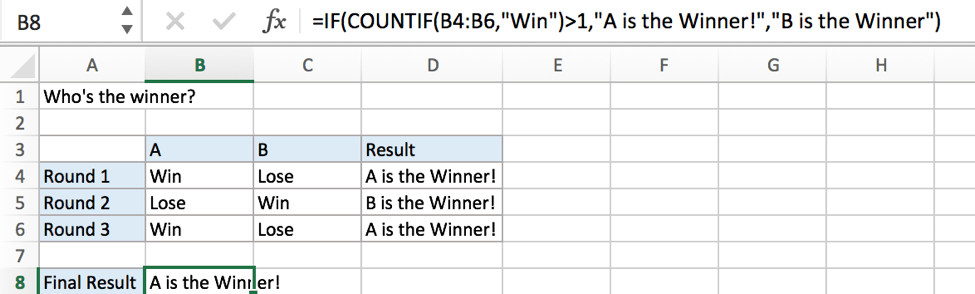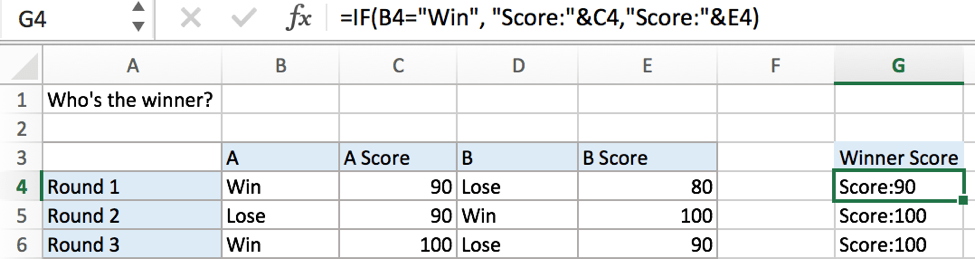# If Cell Equals Certain Text String

IF function is frequently used in Excel worksheet to return you expect “true value” or “false value” based on the result of created logical test. If you want to see if cell equals a certain text string like “Win”, you can build a formula with the help of IF function to return either true result or false result.## FORMULA

To test if cell equals a certain text string, the generic formula is:

=IF(A1=”text”,”true value”,”false value”)

Formula in this example:

=IF(B4=”Win”,”A is the Winner!”,”B is the Winner!”)

## EXPLANATION

In this example, we want to see the results of “A or B who’s the winner” in the three rounds competitions. IF function can handle this case properly.

IF function allows you to create a logical comparison between your value and reference value (for example “A1>0”), and set true value and false value what you expect to return as test results. IF function returns one of the two results based on logical comparison result.

Syntax: IF(logica_test,[value_if_true],[value_if_false])

To test if B4 equals text “Win”, we can directly create a logical comparison B4=“Win”. “Win” should be quoted by double quotes, if missing double quotes, #NAME? error displays instead and it signifies some errors should be corrected in this formula.

In this example, “Win” is detected in B4, logical test result is true.x
How to Show All Named Ranges in Excel

As the result is true, IF function returns true value “A is the Winner!” in C4 properly. On the other side, cell B5 doesn’t equal text “Win”, IF evaluates false value “B is the Winner!” to C5.

In IF, true value and false value can be set as a text, a number or a formula. We set “A is the Winner!” as true result and “B is the Winner” as false result, you can set what you expect to these two values.

More Complicated Example A

To see who is the winner after three rounds of competitions, the winner at least won two among three rounds of competitions, the number of cells “equals “Win”” should be greater than 1. In this case, we can use IF function together with COUNTIF function.More Complicated Example B

You can also change true value or false value to some complicated formula, strings or combinations.### Related Functions

• Excel COUNTIF function
The Excel COUNTIF function will count the number of cells in a range that meet a given criteria. This function can be used to count the different kinds of cells with number, date, text values, blank, non-blanks, or containing specific characters.etc.= COUNTIF (range, criteria)…
• Excel IF function
The Excel IF function perform a logical test to return one value if the condition is TRUE and return another value if the condition is FALSE. The IF function is a build-in function in Microsoft Excel and it is categorized as a Logical Function.The syntax of the IF function is as below:= IF (condition, [true_value], [false_value])….

Related Posts

If Cell is This Value or That Value

IF function is frequently used in Excel worksheet to return you expect “true value” or “false value” based on the result of logical test. If you want to see if a cell is A or B, and if one of ...

If Value is Greater Than A Certain Value

IF function is frequently used in Excel worksheet to return you expect “true value” or “false value” based on the logical test result. If you want to see if a value in one cell is greater than a specific value, ...

If Cell is Not Blank

IF function is frequently used in Excel worksheet to return you expect “true value” or “false value” based on the result of created logical test. If you want to see if a cell is blank or not, and leave some ...

If Cell is Blank

IF function is frequently used in Excel worksheet to return you expect “true value” or “false value” based on the result of created logical test. If you want to see if a cell is blank or not, and leave some ...

If Cell Contains Either Text1 or Text2

IF function is frequently used in Excel worksheet to return “true value” or “false value” based on the logical test result. If you want to see if cell contains certain substring1 like “abc” or substring2 like “def”, and returns true ...

If Cell Contains Certain Text OR Equals Certain Text

IF cell equals certain text IF function is frequently used in Excel worksheet to return “true value” or “false value” based on the logical test result. If you want to test values to see if they equal certain text like ...

Fix #N/A Error For VLOOKUP From Another Sheet

This post will show you how to fix the #N/A error why it occurs when you extract values from another sheet using VLOOKUP function in Excel 2016,2013,2010 or other Excel versions. How can you correct a #N/A error in VLOOKUP ...

How to Average Only Positive or Negative Numbers of a Range

Suppose both positive numbers and negative numbers exist in a table. If we want to know the average of only positive numbers in this table, we can create a formula to get average of all positive numbers with all negative ...

VLOOKUP with Two Lookup Tables

VLOOKUP is one of the key functions among all lookup & reference functions in Excel. Today we will show you the application of VLOOKUP function when there are two lookup tables. EXAMPLE Table1 and table2 record the rates of Y2020 ...

Approximate Match with Multiple Criteria by INDEX & MATCH

In Excel, INDEX function and MATCH function are often used together for returning data from specific position. And MATCH function is one of Excel lookup & reference functions that can return approximate value by setting match type. Above all, through ...

Sidebar